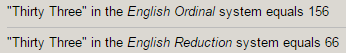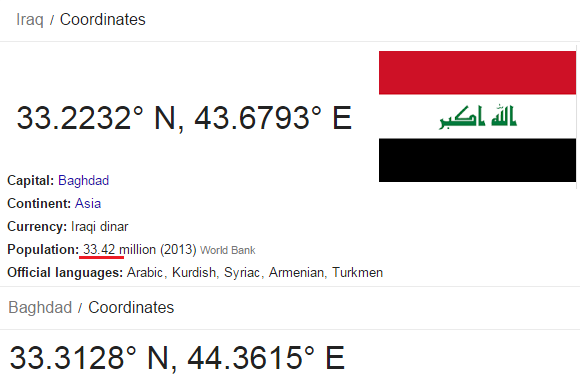CipherJan 3, 2017

Fun little finds

Dimensions of US Dollars156th prime number is 911These dimensions apply for all of the US Dollars

Now check out these nations located at 33° latitudeIt's crazy how in at least 4 of these countries there are ongoing wars at this moment and they are all located at 33° North

Also it's interesting how India is 33° 74°, some people say that entire Christian religion comes from Hinduism, 33 74 being trademark numbers of Christian religion

I was looking at Israel's Wiki page and found something funny4.4 others tells well enough how they feel about non-jews (44 being the kill number basically)74% Jewish people in the nation
Jews write God without O, G-d, 7-4

Israel was founded on 5/14/1948 - 5+1+4+1+9+4+8 = 32 (5+14+1+9+4+8 = 41 the 13th prime number)
Jerusalem = 1+5+9+3+1+1+3+5+4 = 32 (41 with S exception)

Also 5/14 was 135th day of the year in 1948 (leap year), 93 is 135 when converted to Octal numbering system
Israel joined UN on 5/11/1949 = 5+11+1+9+4+9 = 39

Syria - one of 33°North latitude nations (Biggest enemy of Israel)

Syria = 10+7+9+9+1 = 36
Damascus = 4+1+4+1+10+3+3+10 = 36 (Capital city)Iraq - Population - 36 million, but if you just type in google "Iraq" it will give you a preview on the side with outdated number of 33.42 millionThere are 4 countries in Europe that have 33 name Gematria

Belgium = 2+5+3+7+9+3+4 = 33
Belarus = 2+5+3+1+9+3+10 = 33 (S exception)
Finland = 6+9+5+3+1+5+4 = 33
Norway = 5+6+9+5+1+7 = 33Oslo = 15+19+12+15 = 61 (Capital city)
Norway is located at 61°North

France is a nation with +33 dialing code
Officially known as "French Republic" = 77 (Christ = 77) dead at 33